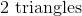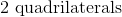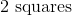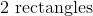# ISEE Lower Level Math : How to find symmetry

## Example Questions

### Example Question #1 : How To Find Symmetry

There is a four sided figure in which none of the lines run parallel to each other. Which of the following could be the appropriate term to describe the figure?

None of these

Parallelogram

Rectangle

Trapezoid

Explanation:

A key characteristic of a rectangle, parallelogram, and trapezoid is the fact that they each have at least one pair of lines that run parallel to each other. The only option that has lines that may not run parallel to each other is the quadrilateral, which must simply have four sides but has no specifications about parallelism.

### Example Question #2 : Lines

If a diagonal is drawn from one corner of a rectangle to the opposite corner, what 2 shapes result?Explanation:

While drawing a line across a rectangle (so that it bisects 2 sides) can result in 2 quadrilaterals, squares, or rectangles, a line drawn from one corner to the furthest corner results in two triangles. Therefore, the correct answer choice is 2 triangles.

### Example Question #3 : Lines

How many lines of symmetry are there in a square?